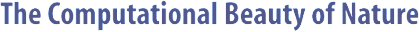Computer Explorations of Fractals, Chaos,Complex Systems, and Adaptation· title page · home* · cover artwork · jacket text · table of contents · the author* · ordering informationBook Contents
 · three themes · part synopses · selected excerpts · all figures from book · quotes from book · glossary from book · bibliography · slide showSource Code
 · overview &documentation · FAQ list* · download source code · java appletsMiscellany
 · news* · reviews & awards · errata · for educators · bibliography (BibTeX format) · other linksGlossary - D

 [ A | B | C | D | E | F | G | H | I | J | K | L | M | N | O | P | Q | R | S | T | U | V | W | X | Y | Z ]

# D

Darwinism     The theory of evolution as proposed by Charles Darwin, which combined variation of inheritable traits with natural selection. After the discovery of the physical mechanism of genetics, this was further refined into neo-Darwinism.

Delta Rule     The perceptron learning rule that specifies that weight changes should be proportional to the product of a weight's input and the error (or delta) term for the perceptron.

Derivative     An expression that characterizes how the output of a function changes as the input is varied. Unlike integrals, derivatives can be calculated in an analytical manner very easily.

Decision Problem     A problem in which all questions take the form ``Is something a member of a particular set?'' and all answers are either ``yes'' or ``no.''

Detector     A sensor that translates the state of a classifier's environment into a message that is suitable for posting to the message list of the classifier system.

Determinant     A quantity of a matrix that characterizes the amount of expansion or contraction that the matrix inflicts on a vector when that vector is multiplied by the matrix.

Deterministic     Occurring in a non-random manner such that the next state of a system depends only on prior states of the system or the environment. Perfect knowledge of previous states implies perfect knowledge of the next state.

Diagonal Matrix     A matrix that has 0 entries along all nondiagonal entries, i.e., only the main diagonal may have non-zero values.

Difference Equation     An equation that describes how something changes in discrete time steps. Numerical solutions to integrals are usually realized as difference equations.

Differential Equation     A description of how something continuously changes over time. Some differential equations can have an analytical solution such that all future states can be known without simulation of the time evolution of the system. However, most can have a numerical solution with only limited accuracy.

Differentiation     The act of calculating a derivative; the inverse operation of calculating an integral.

Diffusion Limited Aggregation     A type of stochastic fractal formed by particles floating about in a random manner until they stick to something solid.

Discrete     Taking only non-continuous values, e.g., Boolean or natural numbers.

Dissipative System     A dynamical system that contains internal friction that deforms the structure of its attractor, thus making motion such as fixed points, limit cycles, quasiperiodicity, and chaos possible. Dissipative systems often have internal structure despite being far from equilibrium, like a whirlpool that preserves its basic form despite being in the midst of constant change.

Diverge     For algorithms or computers, to run forever and never halt; for iterative systems (like the equations for the Mandelbrot set), reaching a state such that all future states explode in size.

Dot Product     The inner product of two vectors.

Dynamical System     A system that changes over time according to a set of fixed rules that determine how one state of the system moves to another state.

Dynamics/Dynamical     Pertaining to the change in behavior of a system over time.

 [ A | B | C | D | E | F | G | H | I | J | K | L | M | N | O | P | Q | R | S | T | U | V | W | X | Y | Z ]Copyright © Gary William Flake, 1998-2002. All Rights Reserved.Last modified: 30 Nov 2002